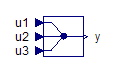Simulation Settings - MapleSim Help

Simulation Settings

In the Simulation Settings tab () you can specify the simulation duration time, the number of plot points, the solver, and other parameters for your model.

The following table describes the parameters available in the Simulation section.  For information on the parameters available in the Advanced Simulation section, see Advanced Simulation Settings.

Parameter

Default

Description

${t}_{d}$

10s

The duration time of the simulation. You can specify any positive value, including floating-point values.

Note: The duration time is not the same as the end time of your simulation. The end time for a simulation is given by td + ts, where ts is the start time for the simulation (see the Advanced Simulation Settings page).

Solver Type

Variable

The type of solver to use for the simulation.

 • Variable: use a variable time step to maintain error tolerances.
 • Fixed: use a fixed time step and disregard integration error.

Note: The fixed step solvers are identical to those used by MapleSim's exported code.

Solver

Variable: CK45 (non-stiff)

Fixed: Euler

DAE solver used during the simulation. The following choices are available when Solver Type is set to Variable.

 • CK45 (semi-stiff): use a semi-stiff DAE solver (ck45 method).
 • RKF45 (non-stiff): use a non-stiff DAE solver (rkf45 method).
 • Rosenbrock (stiff): use a stiff DAE solver (Rosenbrock method).

If your model is complex, you may want to use a stiff DAE solver to reduce the time required to simulate a model.

The following choices are available when Solver Type is set to Fixed.

 • Euler: use a forward Euler solver.
 • Implicit Euler: use an implicit Euler solver (suitable for stiff systems).
 • RK2: use a second-order Runge-Kutta solver.
 • RK3: use a third-order Runge-Kutta solver.
 • RK4: use a fourth-order Runge-Kutta solver.

${\mathrm{ε}}_{\mathrm{abs}}$

$1\cdot {10}^{-5}$

The limit on the absolute error tolerance for a successful integration step if you are using a variable-step solver to run the simulation. You can specify a floating-point value for this option.

${\epsilon }_{\mathrm{rel}}$

$1\cdot {10}^{-5}$

The limit on the relative error tolerance for a successful integration step if you are using a variable-step solver to run the simulation. You can specify a floating-point value for this option.

Step size

0.0010

Uniform size of the sampling periods if you are using a fixed-step solver to run the simulation. You can specify a floating-point value for this option.

Plot Points

2000

Minimum number of points to be plotted in a simulation. The data points are distributed evenly according to the simulation duration value. You can specify a positive integer. Additional points can be added for events (see Plot Events in the Advanced Simulation Settings page).

Note: This option allows you to specify the number of points for display purposes only. The actual number of points used during the simulation may differ from the number of points displayed in the simulation graph.See Also